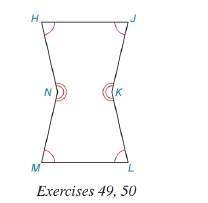Chapter 2.5, Problem 47E### Elementary Geometry for College St...

6th Edition
Daniel C. Alexander + 1 other
ISBN: 9781285195698

#### Solutions

Chapter
Section### Elementary Geometry for College St...

6th Edition
Daniel C. Alexander + 1 other
ISBN: 9781285195698
Textbook Problem
12 views

# For concave hexagon HJKLMN, m ∠ H = y and the measure of reflex angle at N is 2 ( y + 10 ) . Find the measure of the interior angle sat vertex N.(HINT: Note congurences in the figure)To determine

To find:

The measure of the interior angle at vertex N.

Explanation

Given:

The following figure shows the hexagon HJKLMN.

mH=y and measures of reflex angle at N is 2(y+10).

Hexagon has 6 sides.

Formula used:

The formula to calculate the sum of interior angle of an n sided regular polygon is given by,

S=(n2)180°

Calculation:

Substitute 6 for n in the above mentioned formula to obtain the value of S.

S=(62)180°=4×180°=720°

Equation for sum of interior angles of hexagon is as follows.

mH+mJ+mM+mL+mN+mK=720°y+y+

### Still sussing out bartleby?

Check out a sample textbook solution.

See a sample solution

#### The Solution to Your Study Problems

Bartleby provides explanations to thousands of textbook problems written by our experts, many with advanced degrees!

Get Started

#### In Problems 7-12, find the third derivative.

Mathematical Applications for the Management, Life, and Social Sciences

#### In Exercises 516, evaluate the given quantity. log4116

Finite Mathematics and Applied Calculus (MindTap Course List)

#### The slope of the tangent line to y = x3 at x = 2 is: 18 12 6 0

Study Guide for Stewart's Single Variable Calculus: Early Transcendentals, 8th

#### True or False: , for –π ≤ t ≤ π, is smooth.

Study Guide for Stewart's Multivariable Calculus, 8th